02.03.2021 г.

When do we stop the recognition process in a video stream?

Today we’d like to delve into a very interesting problem that we’ve been working on since we started researching document recognition in a video stream — the problem of finding the optimal stopping point.

Figure 1. Images of ID-document text field in video frames

As many of you know, one of the main products of Smart Engines is an identification document recognition system, Smart IDReader, that is compatible with servers, desktops, and mobile devices. It’s common that document recognition on mobile devices has to happen in an autonomous mode. More often than not we are not able to control the circumstances and the quality of the video, meanwhile, the cost of recognition errors, especially when working with identification documents, is quite high. But we shouldn’t forget that we have a serious advantage: we are able to use not just one image, but a sequence of images in a video stream (see Figure 1) to improve the recognition accuracy.

When using a set of text field frames, there will be two major points we’ll need to address. The first question arising is how we would combine the information from different frames. Should we integrate the original frames in order to get one image of “higher” quality, or should we pick the best image (but we are not guaranteed to get an image that we’ll meet all our requirements)? Or, maybe, we should do the text field recognition for each image, and then choose the best option (according to which criteria though?), or we could integrate the recognized text fields, etc. We find the last approach to be the most effective (recognition of all the images and integration of the results), although the optimal approach might differ depending on the recognition engine and other possible task characteristics.

The second problem (it occurs independently from the first one) is when to stop the recognition process. In other words, what criteria do we use to decide that we can stop the image collection and that the material accumulated up to a certain point is sufficient for our purposes and the combined result can be considered as final? How do we compare these criteria and is there an optimal one?

This stopping problem will be the main focus of our article.

What are we trying to achieve?

Text field recognition in a video stream with a result that improves every time we get new information can be viewed as an anytime algorithm. An anytime algorithm finds better and better solutions the longer it keeps running and can be interrupted at any given moment. Expected performance profiles are a great quality visualization tool for such algorithms. They are the graphs that show the relationship between the result quality that can be measured in different ways and the computation time.

Figure 2. The expected performance profiles for text field recognition in a video stream (the lower — the better).

The dotted black line shows the per-frame quality, the black solid line — the results of per-frame integration. The orange line shows what we expect to happen when we apply a “good” stopping rule.

Figure 2 demonstrates the performance profiles for text field recognition. They show the relationship between an average error (in terms of the Levenshtein distance to the correct answer) and the number of processed frames. The black graphs are the results of Tesseract v4.0.0 recognition on MIDV-500 dataset. We can see that the recognized material integration decreases the error quantity significantly (which is not surprising at all).

What is a “good” stopping rule then? Since we reasonably expect that the longer we run the process, the better the result will be on average, it would be great if the stopping rule doesn’t stop for a longer period of time in some video streams if there is a chance to minimize errors, and in other video streams it could stop earlier if the result is good enough already, or there is no way to improve it. When this principle is applied, with the same average quality of the integrated result we might possibly need fewer images that need to be recognized, or vice versa — when working with the same average number of images, the integrated result quality might be better. In other words, we have to be aware that the stopping rule is not just about time minimization but about quality maximization as well (on average, over the same period of time).

Let’s imagine we are trying to find the stopping rule in the following form: after we processed another frame and got an integrated recognition result, we calculate some characteristic and perform thresholding based on it. If it’s lower than the threshold, we stop, if it’s not, we continue the process. When we use the fixed stopping rule but change the threshold, we’ll get the performance profile as well. The only difference is that the horizontal axis will indicate not the exact number of processed frames, but an average number (see the orange graph in Figure 2). The lower this graph runs, the more effective the stopping rule. The original profile of the integrated result can be viewed as a performance profile of a trivial stopping rule — when we produce a threshold cut-off of just the number of processed frames.

What does the theory say?

The problems of finding the optimal stopping strategies are not novel in the world of mathematical statistics and have been a subject of research for a while now. The secretary problem (Boris Abramovich Berzovsky has been studying it extensively, for example) and the house-selling problem are arguably the most known problems of this kind. Without going deep into theory, let’s briefly define the video stream recognition problem as a problem of optimal stopping.

Let’s denote an integrated recognition error at stageof the process as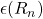. We assume that if we stop at stage, the loss function will appear as follows: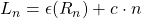, where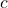— some pre-defined relative cost of each observation. The stopping problem can be defined as determining a random variable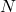— the stopping time that depends on the input data (in some sources the variableis called Markov moment or Markov time) and when applied it minimizes expected loss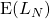.

Monotone problems

In certain circumstances we can clearly determine the optimal stopping rule when working with similar problems. Monotone stopping problems are a good example. The problem is considered to be monotone if the loss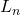at some stageis not higher than expected loss at the next stage, then this will be true for all future stages as well. In other words,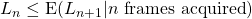implies. The optimal stopping rule for monotone problems will be the so-called myopic rule: stop at the stage when the following requirement is met.

Let’s suppose that we have a monotone stopping problem. If we write the myopic rule in terms of our function, it means we have to stop when the following condition is met: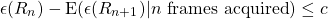It all sounds great but in order to apply this rule, we need to be able to estimate not only the ‘correctness’ of the current result, but the expected correctness of the following result as well and to do so during runtime. This is not an easy task (not to mention the monotone problem requirements that have to be met). Is there a way to apply this rule without directly estimating the “correctness” of the result? We could try to find an upper bound for the left part of the inequality.

How can we use the myopic rule?

Let’s assume that the functionequals the distance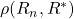from the integrated recognition result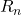to “the ideal result”, and just like any other distance, it satisfies triangle inequality. The above-mentioned Levenshtein distance satisfies the triangle inequality, as well as a simple “correct/incorrect” kind of function (if we agree thatequals zero when the result is correct, and it equals a constant when the result is incorrect). According to the triangle inequality, the left part of our stopping rule requirement is not higher than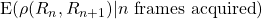— the expected distance from the current integrated result to the next one.

Let’s assume that our algorithm for inter-frame integration of the recognition results works in such a way that the distance between the consequent integrated results don’t increase over time (which means we’ll view the sequence of integrated results as some “converging” process, not necessarily leading to the correct result though). In this case if the expected distance from the current result to the next one is getting lower than the threshold, then two things are true. First, the stopping criterion of the myopic rule is met (since its left side limited to this distance). And secondly, the problem becomes monotone: the expected distance to the next result is not getting higher at the next stage, which means it will stay lower than the threshold and the myopic rule requirement will be met again.

This means that if we expect that the distances between the consequent integrated results do not increase on average, we need to determine the stopping point by performing thresholding of the expected distance from the current result to the next one, therefore approximating the optimal rule. We need to understand that this rule is not optimal anymore (the “true” optimal rule could’ve stopped earlier), but at least we won’t stop too early.

We can estimate the expected distance from the current integrated result to the next one in various ways. For example, if we use the Levenshtein distance as a metric for results, then even the distance between two last recognition results would be a good approximation. A different approach would be to simulate a possible next observation (for example, based on the previous observations), to perform “blank” integrations and to calculate an average distance to these predicted results.

Figure 3. Performance profile comparison for different stopping rules.

Figure 3 exhibits the performance profiles for a few different stopping rules.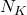is the above-mentioned “trivial rule” that performs thresholding of a certain number of processed observations.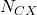and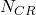— thresholding of the largest cluster of identical frame-by-frame results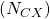and integrated results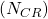.is a rule described in this article that estimates the expected distance to the next result using simulation and “blank” integrations.

In lieu of conclusion

The goal of this article was to show that there are a number of challenging problems when it comes to document recognition in a video stream. These problems are not just computer vision related ones. They come from different research fields. While we reviewed the problem of finding an optimal stopping point only in its simplest form, the most exciting part starts when we want to take into account not just the number of frames, but the real time of processing, for example. We hope that you found this read entertaining!

This piece is written based on the article “On optimal stopping strategies for text recognition in a video stream”, K. Bulatov, N. Razumnyi, V. V. Arlazarov, IJDAR 22, 303-314 (2019) 10.1007/s10032-019-00333-0.

If the reader is interested in the theory of optimal stopping, e.g. the proof that the myopic rule is optimal for monotone problems, we highly recommend to read the published course Optimal Stopping and Applications (Thomas Ferguson, UCLA).

A few more good sources on this subject:

Chow Y.S., Siegmund D. Great expectations: The theory of optimal stopping, Houghton Mifflin, 1971, 139 p.
Ferguson T.S., Hardwick T.S. Stopping rules for proofreading, Journal of Applied Probability., V. 26, N. 2, 1989, p. 303-313.
Ferguson T.S. Mathematical statistics: a decision theoretic approach. Academic press, 1967, 396 p.